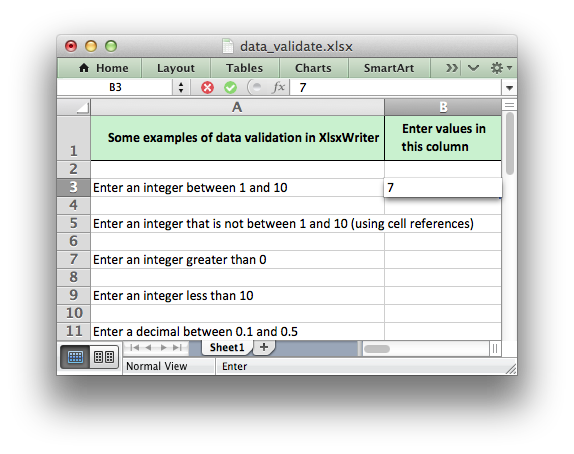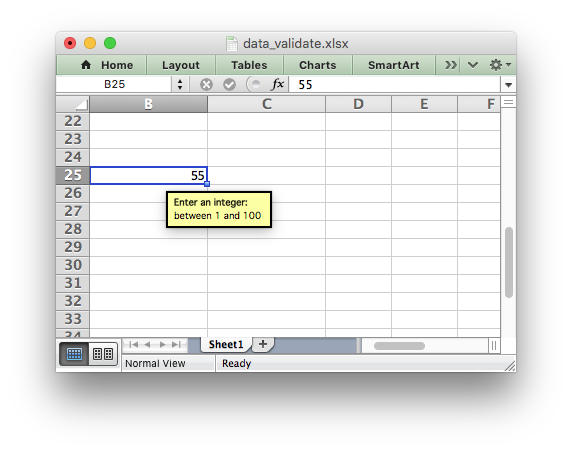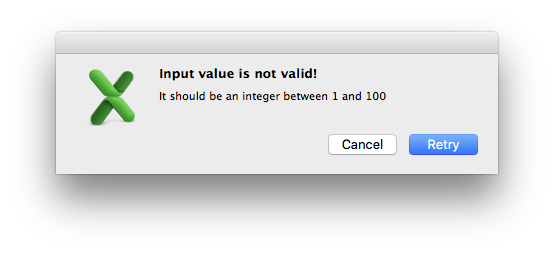# Working with Data Validation

Data validation is a feature of Excel which allows you to restrict the data that a user enters in a cell and to display associated help and warning messages. It also allows you to restrict input to values in a dropdown list.

A typical use case might be to restrict data in a cell to integer values in a certain range, to provide a help message to indicate the required value and to issue a warning if the input data doesn’t meet the stated criteria. In XlsxWriter we could do that as follows:

```worksheet.data_validation('B25', {'validate': 'integer',
'criteria': 'between',
'minimum': 1,
'maximum': 100,
'input_title': 'Enter an integer:',
'input_message': 'between 1 and 100'})
```If the user inputs a value that doesn’t match the specified criteria an error message is displayed:For more information on data validation see the Microsoft support article “Description and examples of data validation in Excel”: http://support.microsoft.com/kb/211485.

The following sections describe how to use the `data_validation()` method and its various options.

## data_validation()

The `data_validation()` method is used to construct an Excel data validation.

The data validation can be applied to a single cell or a range of cells. As usual you can use A1 or Row/Column notation, see Working with Cell Notation.

With Row/Column notation you must specify all four cells in the range: `(first_row, first_col, last_row, last_col)`. If you need to refer to a single cell set the last_ values equal to the first_ values. With A1 notation you can refer to a single cell or a range of cells:

```worksheet.data_validation(0, 0, 4, 1, {...})
worksheet.data_validation('B1',       {...})
worksheet.data_validation('C1:E5',    {...})
```

The options parameter in `data_validation()` must be a dictionary containing the parameters that describe the type and style of the data validation. The main parameters are:

 `validate` `criteria` `value` `minimum` `source` `maximum` `ignore_blank` `dropdown` `input_title` `input_message` `show_input` `error_title` `error_message` `error_type` `show_error` `multi_range`

These parameters are explained in the following sections. Most of the parameters are optional, however, you will generally require the three main options `validate`, `criteria` and `value`:

```worksheet.data_validation('A1', {'validate': 'integer',
'criteria': '>',
'value': 100})
```

### validate

The `validate` parameter is used to set the type of data that you wish to validate:

```worksheet.data_validation('A1', {'validate': 'integer',
'criteria': '>',
'value': 100})
```

It is always required and it has no default value. Allowable values are:

```integer
decimal
list
date
time
length
custom
any
```
• integer: restricts the cell to integer values. Excel refers to this as ‘whole number’.
• decimal: restricts the cell to decimal values.
• list: restricts the cell to a set of user specified values. These can be passed in a Python list or as an Excel cell range.
• date: restricts the cell to date values specified as a datetime object as shown in Working with Dates and Time or a date formula.
• time: restricts the cell to time values specified as a datetime object as shown in Working with Dates and Time or a time formula.
• length: restricts the cell data based on an integer string length. Excel refers to this as ‘Text length’.
• custom: restricts the cell based on an external Excel formula that returns a `TRUE/FALSE` value.
• any: is used to specify that the type of data is unrestricted. It is mainly used for specifying cell input messages without a data validation.

### criteria

The `criteria` parameter is used to set the criteria by which the data in the cell is validated. It is almost always required except for the `list`, `custom` and `any` validate options. It has no default value:

```worksheet.data_validation('A1', {'validate': 'integer',
'criteria': '>',
'value': 100})
```

Allowable values are:

 `between` `not between` `equal to` `==` `not equal to` `!=` `greater than` `>` `less than` `<` `greater than or equal to` `>=` `less than or equal to` `<=`

You can either use Excel’s textual description strings, in the first column above, or the more common symbolic alternatives. The following are equivalent:

```worksheet.data_validation('A1', {'validate': 'integer',
'criteria': '>',
'value': 100})

worksheet.data_validation('A1', {'validate': 'integer',
'criteria': 'greater than',
'value': 100})
```

The `list`, `custom` and `any` validate options don’t require a `criteria`. If you specify one it will be ignored:

```worksheet.data_validation('B13', {'validate': 'list',
'source': ['open', 'high', 'close']})

worksheet.data_validation('B23', {'validate': 'custom',
'value': '=AND(F5=50,G5=60)'})
```

### value, minimum, source

The `value` parameter is used to set the limiting value to which the `criteria` is applied. It is always required and it has no default value. You can also use the synonyms `minimum` or `source` to make the validation a little clearer and closer to Excel’s description of the parameter:

```# Using 'value'.
worksheet.data_validation('A1', {'validate': 'integer',
'criteria': 'greater than',
'value': 100})

# Using 'minimum'.
worksheet.data_validation('B11', {'validate': 'decimal',
'criteria': 'between',
'minimum': 0.1,
'maximum': 0.5})

# Using 'source'.
worksheet.data_validation('B10', {'validate': 'list',
'source': '=\$E\$4:\$G\$4'})

# Using 'source' with a string list.
worksheet.data_validation('B13', {'validate': 'list',
'source': ['open', 'high', 'close']})
```

Note, when using the `list` validation with a list of strings, like in the last example above, Excel stores the strings internally as a Comma Separated Variable string. The total length for this string, including commas, cannot exceed the Excel limit of 255 characters. For longer sets of data you should use a range reference like the prior example above. Also any double quotes in strings like `'"Hello"'` must be double quoted like this `'""Hello""'`.

### maximum

The `maximum` parameter is used to set the upper limiting value when the `criteria` is either `'between'` or `'not between'`:

```worksheet.data_validation('B11', {'validate': 'decimal',
'criteria': 'between',
'minimum': 0.1,
'maximum': 0.5})
```

### ignore_blank

The `ignore_blank` parameter is used to toggle on and off the ‘Ignore blank’ option in the Excel data validation dialog. When the option is on the data validation is not applied to blank data in the cell. It is on by default:

```worksheet.data_validation('B5', {'validate': 'integer',
'criteria': 'between',
'minimum': 1,
'maximum': 10,
'ignore_blank': False,
})
```

### input_title

The `input_title` parameter is used to set the title of the input message that is displayed when a cell is entered. It has no default value and is only displayed if the input message is displayed. See the `input_message` parameter below.

The maximum title length is 32 characters.

### input_message

The `input_message` parameter is used to set the input message that is displayed when a cell is entered. It has no default value:

```worksheet.data_validation('B25', {'validate': 'integer',
'criteria': 'between',
'minimum': 1,
'maximum': 100,
'input_title': 'Enter an integer:',
'input_message': 'between 1 and 100'})
```

The input message generated from the above example is:The message can be split over several lines using newlines. The maximum message length is 255 characters.

### show_input

The `show_input` parameter is used to toggle on and off the ‘Show input message when cell is selected’ option in the Excel data validation dialog. When the option is off an input message is not displayed even if it has been set using `input_message`. It is on by default.

### error_title

The `error_title` parameter is used to set the title of the error message that is displayed when the data validation criteria is not met. The default error title is ‘Microsoft Excel’. The maximum title length is 32 characters.

### error_message

The `error_message` parameter is used to set the error message that is displayed when a cell is entered. The default error message is “The value you entered is not valid. A user has restricted values that can be entered into the cell.”. A non-default error message can be displayed as follows:

```worksheet.data_validation('B27', {'validate': 'integer',
'criteria': 'between',
'minimum': 1,
'maximum': 100,
'input_title': 'Enter an integer:',
'input_message': 'between 1 and 100',
'error_title': 'Input value not valid!',
'error_message': 'It should be an integer between 1 and 100'})
```

Which give the following message:The message can be split over several lines using newlines. The maximum message length is 255 characters.

### error_type

The `error_type` parameter is used to specify the type of error dialog that is displayed. There are 3 options:

```'stop'
'warning'
'information'
```

The default is `'stop'`.

### show_error

The `show_error` parameter is used to toggle on and off the ‘Show error alert after invalid data is entered’ option in the Excel data validation dialog. When the option is off an error message is not displayed even if it has been set using `error_message`. It is on by default.

### multi_range

The `multi_range` option is used to extend a data validation over non-contiguous ranges.

It is possible to apply the data validation to different cell ranges in a worksheet using multiple calls to `data_validation()`. However, as a minor optimization it is also possible in Excel to apply the same data validation to different non-contiguous cell ranges.

This is replicated in `data_validation()` using the `multi_range` option. The range must contain the primary range for the data validation and any others separated by spaces.

For example to apply one data validation to two ranges, `'B3:K6'` and `'B9:K12'`:

```worksheet.data_validation('B3:K6', {'validate': 'integer',
'criteria': 'between',
'minimum': 1,
'maximum': 100,
'multi_range': 'B3:K6 B9:K12'})
```

## Data Validation Examples

Example 1. Limiting input to an integer greater than a fixed value:

```worksheet.data_validation('A1', {'validate': 'integer',
'criteria': '>',
'value': 0,
})
```

Example 2. Limiting input to an integer greater than a fixed value where the value is referenced from a cell:

```worksheet.data_validation('A2', {'validate': 'integer',
'criteria': '>',
'value': '=E3',
})
```

Example 3. Limiting input to a decimal in a fixed range:

```worksheet.data_validation('A3', {'validate': 'decimal',
'criteria': 'between',
'minimum': 0.1,
'maximum': 0.5,
})
```

Example 4. Limiting input to a value in a dropdown list:

```worksheet.data_validation('A4', {'validate': 'list',
'source': ['open', 'high', 'close'],
})
```

Example 5. Limiting input to a value in a dropdown list where the list is specified as a cell range:

```worksheet.data_validation('A5', {'validate': 'list',
'source': '=\$E\$4:\$G\$4',
})
```

Example 6. Limiting input to a date in a fixed range:

```from datetime import date

worksheet.data_validation('A6', {'validate': 'date',
'criteria': 'between',
'minimum': date(2013, 1, 1),
'maximum': date(2013, 12, 12),
})
```

Example 7. Displaying a message when the cell is selected:

```worksheet.data_validation('A7', {'validate': 'integer',
'criteria': 'between',
'minimum': 1,
'maximum': 100,
'input_title': 'Enter an integer:',
'input_message': 'between 1 and 100',
})
```